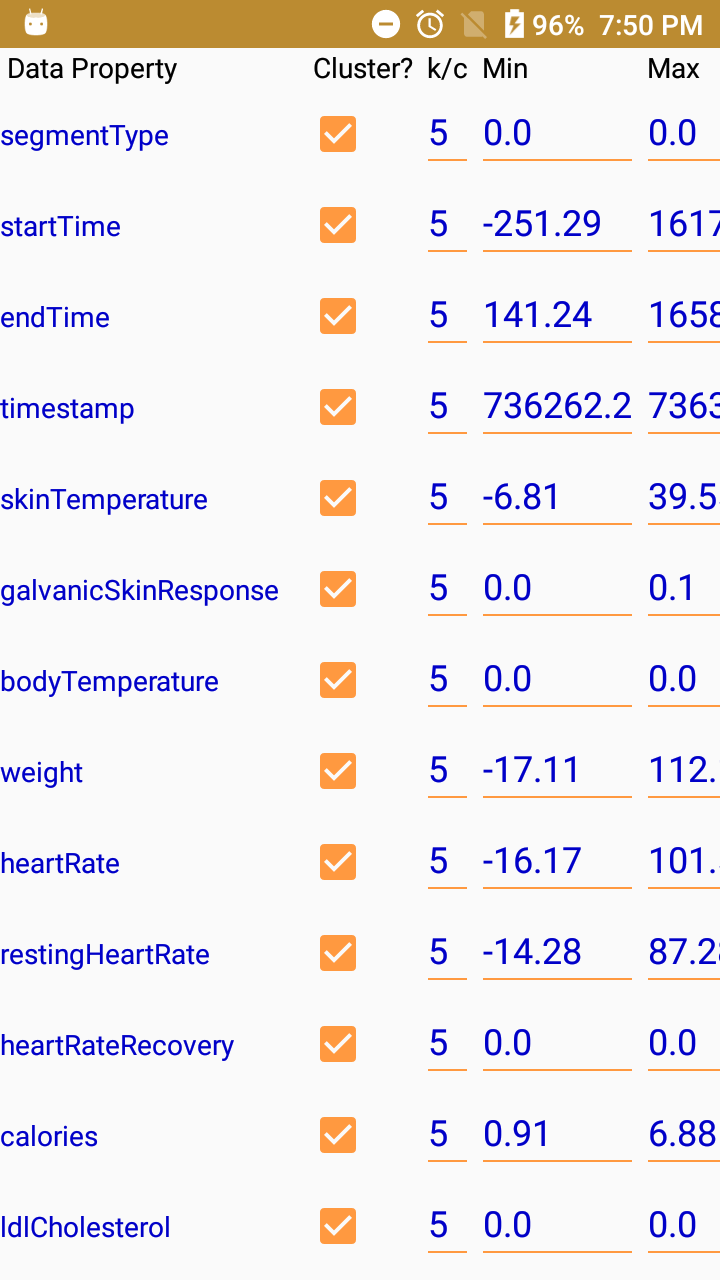DATIL (DATatypes with Imprecision Learner) is a software that automatically learns fuzzy datatypes for fuzzy ontologies from different types of inputs. Datil implements several unsupervised clustering algorithms: k-means, fuzzy c-means, and mean-shift.

For each data property in an ontology with a numerical range (or with assertions involving numerical values), Datil collects an array of real numbers corresponding to the values of the property for different individuals. A clustering algorithm provides a set of centroids from these array of values. These centroids are used as the parameters to build fuzzy membership functions partitioning the domain (Left figure). Graphic User Interface is shown in the right figure.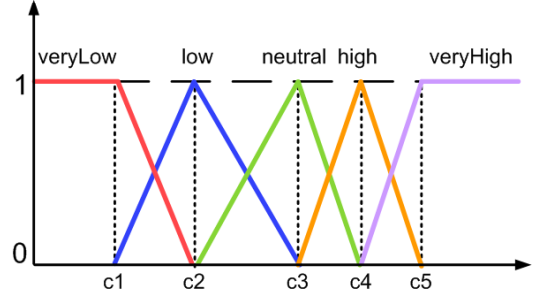###### Some fuzzy membership functions built from the centroids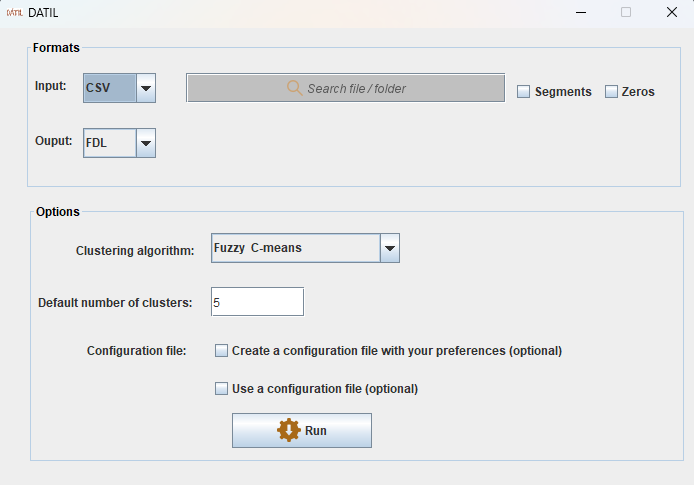##### Datil supports:

Input formats: .owl, .fdl, and .csv.
Output format: .owl, and .fdl.

##### Datil permits configuration options:

– The input and output formats.
– The input file. The output file is not a parameter; Datil uses the same filename (with a different filename extension if there is a format change).
– The selected clustering algorithm.
– The number of clusters (only for k-means and fuzzy c-means) for all the properties, or a different number for each property.
– The properties for which to learn the fuzzy datatypes.
– Use of zeros (only for .csv files): zero values can be either taken into account or skipped (in practice, they are often used just to represent empty data).
– Use of segments (only for .csv input files), i.e., the first column can have a special meaning classifying each row as belonging to a different category.
– The range of values [Min, Max] for each data property. In general, Datil uses some strategies to obtain automatically the fuzzy datatype range [k1, k2] for each data property of the input file, but this automatic values can be customized by the user; for example, if there are outliers, or to reduce/amplify the range that will be considered to compute the fuzzy datatypes.

It is also possible to use a configuration file to select a subset of the data properties and/or select a different number of clusters for each of them if the clustering algorithm is not mean-shift. Right figure shows how Datil supports the creation of the file by making its syntax transparent to the user. Thanks to the configuration file the user does not need to repeat the selection in future executions. If the system does not find a configutation file, it runs with the default values.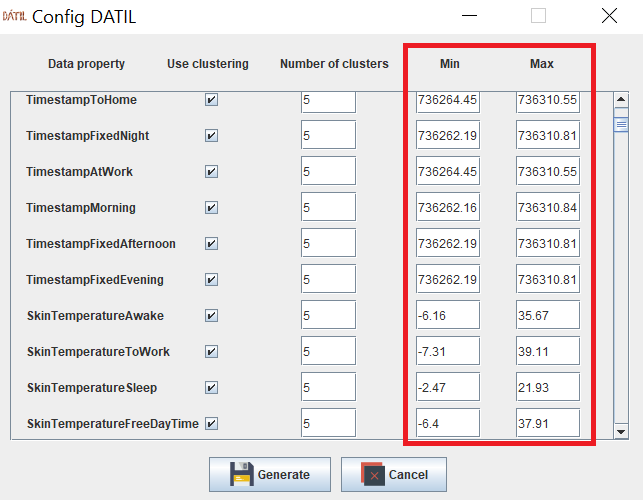###### GUI to create a configuration file in Datil

Datil app is developed on Android platform, some screenshot are visible in the Figure a) and b).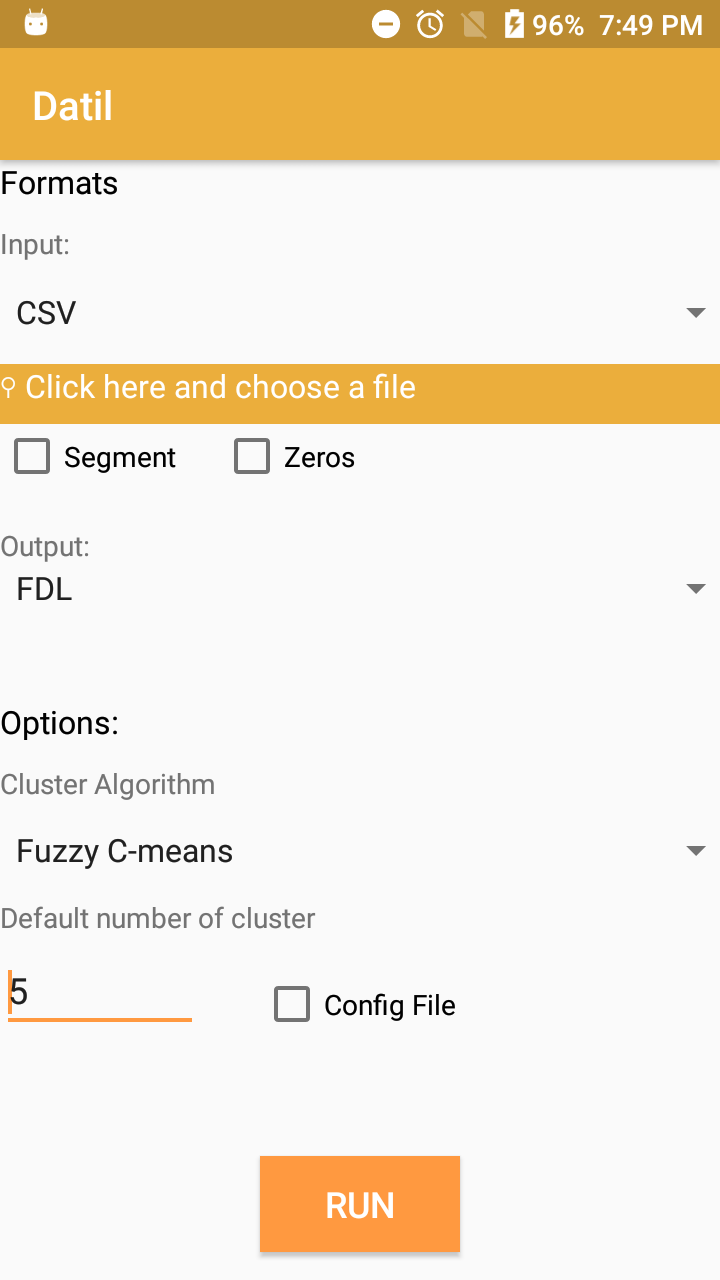###### a)Datil app: main interface